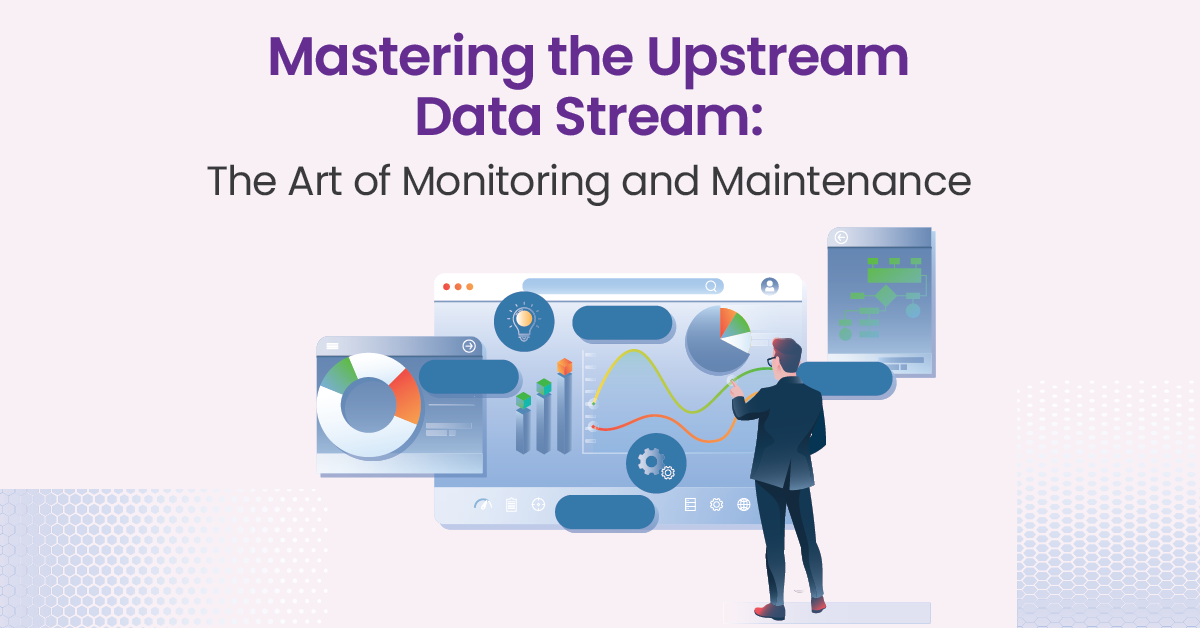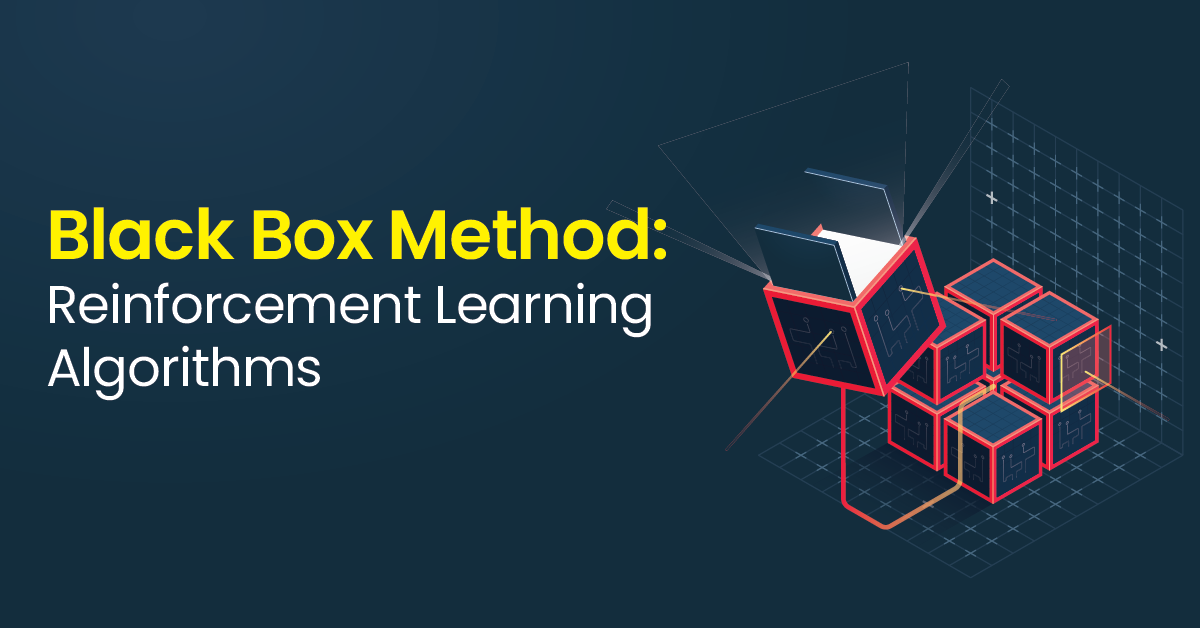Home / Blog / Machine Learning / Stochastic Gradient Descent: A Comprehensive Guide

# Stochastic Gradient Descent: A Comprehensive Guide

• November 11, 2023
• 2720
• 94### Meet the Author : Mr. Bharani Kumar

Bharani Kumar Depuru is a well known IT personality from Hyderabad. He is the Founder and Director of Innodatatics Pvt Ltd and 360DigiTMG. Bharani Kumar is an IIT and ISB alumni with more than 17 years of experience, he held prominent positions in the IT elites like HSBC, ITC Infotech, Infosys, and Deloitte. He is a prevalent IT consultant specializing in Industrial Revolution 4.0 implementation, Data Analytics practice setup, Artificial Intelligence, Big Data Analytics, Industrial IoT, Business Intelligence and Business Management. Bharani Kumar is also the chief trainer at 360DigiTMG with more than Ten years of experience and has been making the IT transition journey easy for his students. 360DigiTMG is at the forefront of delivering quality education, thereby bridging the gap between academia and industry.

Table of Content

## Introduction

Before we explore stochastic gradient descent, it's essential to grasp the fundamentals of the gradient descent algorithm. Gradient descent is an optimization method employed to minimize a cost or loss function, and it is widely used in machine learning for model training. The core concept of gradient descent is to iteratively adjust the model's parameters in the direction of the steepest decrease in the loss function.

The algorithm begins with an initial set of parameters and calculates the gradient of the loss function concerning these parameters. The gradient indicates the direction of the quickest increase in loss. To minimize the loss, we move in the opposite direction of the gradient by taking small steps, typically referred to as the learning rate.

The algorithm begins with an initial set of parameters and calculates the gradient of the loss function concerning these parameters. The gradient indicates the direction of the quickest increase in loss. To minimize the loss, we move in the opposite direction of the gradient by taking small steps, typically referred to as the learning rate.

Become a Data Science Course expert with a single program. Go through 360DigiTMG's Data Science Course Course in Hyderabad. Enroll today!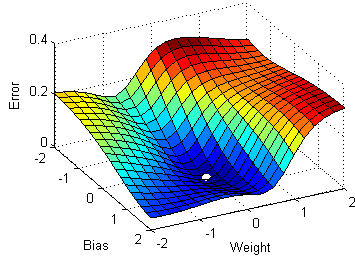Stochastic Gradient Descent is a variation of gradient descent that aims to expedite the optimization process by using a single data point or a small subset of data points to compute the gradient at each step. This introduces an element of randomness in the parameter updates. Let's break down the steps involved in SGD: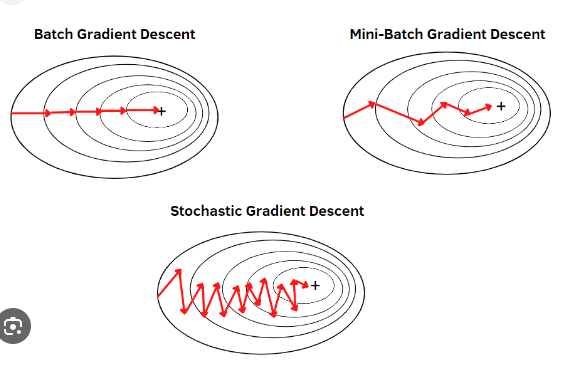### Visualizing Gradient Descent and its types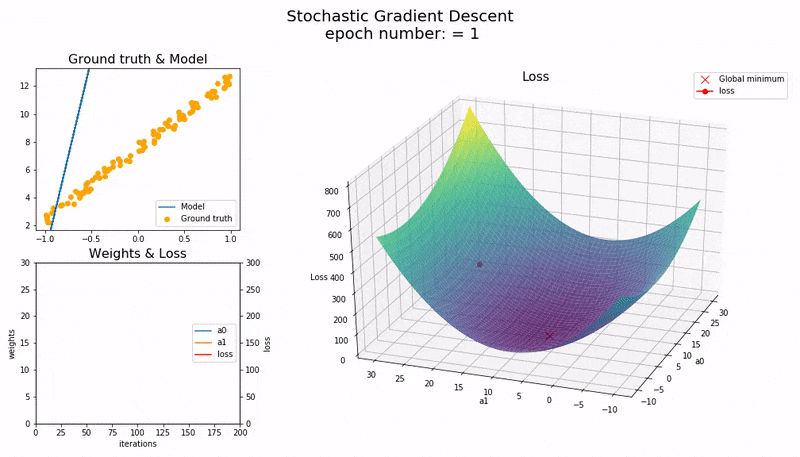## Parameters of SGD

Stochastic Gradient Descent (SGD) is a derivative of the gradient descent optimization algorithm. It differentiates itself by updating the model parameters for each individual training example, as opposed to batch gradient descent, which employs the entire dataset to compute gradients. In SGD, the parameters are updated based on a randomly chosen training instance, which can lead to quicker convergence due to more frequent updates, albeit with increased noise. However, this approach can also introduce oscillations in the cost function, given the stochastic nature of these updates. The parameter update rule for SGD is represented as θ = θ - α · ∇Ji(θ), where θ signifies the parameter vector, α is the learning rate, and ∇Ji(θ) corresponds to the gradient of the cost function J concerning θ, which is computed for a single, randomly selected training example. These distinctive characteristics make SGD a valuable optimization tool in machine learning, offering the potential for faster convergence and adaptability to large datasets.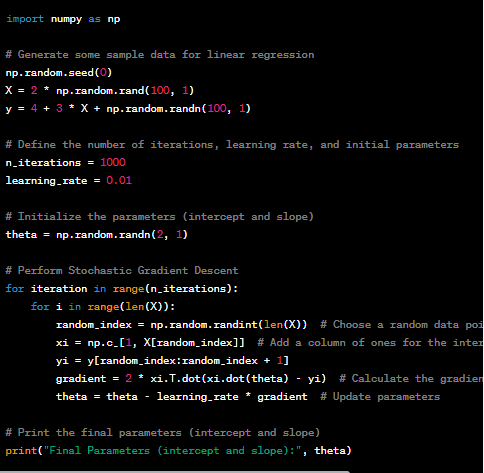Gradient Descent (SGD) for linear regression. This code is for educational purposes and is not optimized for production use. It demonstrates the basic concept of how SGD works in the context of linear regression.

In this code:

• We generate some sample data for a linear regression problem.
• We set the number of iterations, learning rate, and initial parameters (intercept and slope).
• We perform stochastic gradient descent for the specified number of iterations. In each iteration, we randomly select one data point(random_index) and update the parameters using the gradient computed for that data point.
• Finally, we print the learned parameters, which should be close to the true values (4 and 3 in this case).

Random Shuffling: Randomly shuffle the dataset to introduce stochasticity.

Iterative Update: For each data point or a mini-batch of data points, calculate the gradient of the loss function concerning the current parameters.

Parameter Update: Update the parameters by taking a step in the direction of the negative gradient with a specified learning rate.

Repeat: Continue this process for a fixed number of iterations or until convergence criteria are met.

Faster Convergence: Since each update is based on a small subset of data, SGD often converges faster than gradient descent.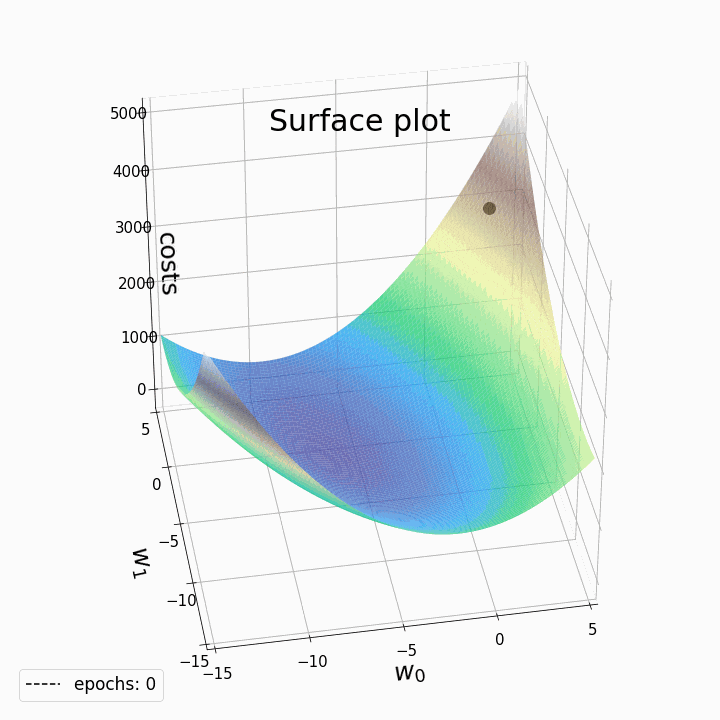Escape Local Minima: The randomness in updates allows SGD to escape local minima and find better solutions. The concept of escaping local minima is crucial in training complex neural networks, where the cost landscape is high-dimensional and full of numerous local minima. SGD's stochasticity, coupled with a properly tuned learning rate, provides a more dynamic exploration process, helping the optimization process converge to better solutions, which may not have been attainable using deterministic gradient-based methods. This inherent ability to escape local minima contributes significantly to the effectiveness of SGD in training deep learning models.Scalability: SGD can handle large datasets that may not fit in memory by processing them in smaller batches.The scalability of SGD refers to its ability to efficiently handle large datasets and high-dimensional model parameters. This efficiency is a result of its update mechanism, where only a random subset of the training data, known as a mini-batch, is used at each iteration. This mini-batch processing significantly reduces the computational and memory requirements, making SGD well-suited for big data applications..SGD's scalability is a key advantage that enables efficient training on large datasets and the utilization of parallel processing for faster convergence, making it a practical and versatile optimization algorithm for machine learning tasks of various scales and complexities.

Regularization Effect: The noise in updates acts as implicit regularization, preventing overfitting.One common form of regularization in machine learning is L2 regularization, which encourages smaller parameter values. In the context of SGD, this effect arises naturally. The frequent updates to model parameters with small learning rates can help prevent them from becoming overly large, thus acting as a form of weight decay or L2 regularization.

Data Science, AI and Data Engineering is a promising career option. Enroll in Data Science course in Chennai Program offered by 360DigiTMG to become a successful Career.

### Challenges of Stochastic Gradient Descent

While SGD offers various benefits, it also comes with challenges:

Noisy Updates: The randomness can introduce noisy updates that hinder convergence in some cases.

Learning Rate Tuning: The learning rate is a critical hyperparameter in SGD, and finding the right value can be challenging.

Convergence Criteria: Determining when to stop training is not always straightforward, and early stopping may lead to suboptimal results.

Sensitivity to Initialization: The choice of initial parameters can affect convergence.

### Learning Rate Schedules

The learning rate in SGD is a crucial hyperparameter that influences the algorithm's behavior. Using a fixed learning rate may not be ideal, as it can lead to slow convergence or instability. Learning rate schedules or strategies adjust the learning rate dynamically during training to address these issues.

• Fixed Learning Rate: This maintains a constant learning rate throughout training. It's simple but may require manual tuning.
• Step Decay: The learning rate is reduced at predefined epochs or steps. This can help fine-tune the learning rate during training.
• Exponential Decay: This strategy causes the learning rate to decrease exponentially over time, often resulting in faster convergence.

Stochastic Gradient Descent typically operates on a single data point at a time (pure SGD) or a small random subset (mini-batch SGD). Mini-batch SGD is the most commonly used variant in deep learning. It strikes a balance between the efficiency of pure SGD and the stability of full-batch gradient descent.

In mini-batch SGD, the training dataset is divided into smaller batches, each containing a fixed number of data points. At each iteration, a mini-batch is randomly selected, and the gradient is calculated using only the data points within that mini-batch. The parameters are then updated based on this mini-batch gradient.

The choice of mini-batch size can significantly impact the training process. A smaller mini-batch size introduces more stochasticity but can lead to faster convergence. A larger mini-batch size provides a more stable gradient estimate but may require more memory.

### Variations of SGD

SGD has given rise to several variations and improvements, each designed to address specific optimization challenges. Here are a few notable ones:

Momentum: The Momentum algorithm introduces a moving average of past gradients, which smooths out noisy updates, aiding the optimization process.

Nesterov Accelerated Gradient (NAG): NAG is an enhancement over Momentum. It calculates the gradient at the lookahead position rather than the current position, resulting in even better convergence.

RMSprop: RMSprop is another adaptive learning rate method that mitigates the diminishing learning rate issue observed in AdaGrad.

Adam: The Adam optimizer combines the benefits of Momentum and RMSprop, making it one of the most popular optimization algorithms for training deep neural networks.

L-BFGS: The Limited-memory Broyden-Fletcher-Goldfarb-Shanno (L-BFGS) is a quasi-Newton optimization method used in scenarios where batch training is feasible and a more precise gradient is required.

Earn yourself a promising career in Data Science by enrolling in Data Science Course in Bangalore offered by 360DigiTMG.

### Convergence and Hyperparameter Tuning

Training deep learning models with SGD is an iterative and often time-consuming process. Achieving convergence may require a substantial number of iterations, and tuning hyperparameters can be challenging.

Here are some tips for efficient training:

Early Stopping: Monitor the loss on a validation dataset and halt training when it begins to increase, indicating overfitting.

Learning Rate Finder: Utilize learning rate range tests to determine a suitable learning rate before training.

Grid Search: Conduct a grid search to fine-tune hyperparameters, including learning rate, mini-batch size, and regularization strength.

Regularization: Employ techniques like dropout, weight decay, and batch normalization to improve model generalization.

Visualization: Visualize the learning process to gain insights into the training dynamics and potential issues.

## Conclusion

In conclusion, Stochastic Gradient Descent (SGD) is a fundamental optimization algorithm in the realm of machine learning and deep learning. Its stochastic nature, which involves randomly selecting a subset of training examples at each iteration, makes it highly efficient, particularly when dealing with vast datasets. While it may not always converge to the global minimum, SGD's random sampling can help escape local minima and foster a degree of robustness in the optimization process. To harness its full potential, careful tuning of hyperparameters such as the learning rate and batch size is necessary, and practitioners often turn to variants like Mini-batch Gradient Descent, Momentum, RMSprop, and Adam to enhance its performance. Overall, SGD's versatility, efficiency, and ability to handle large-scale data make it an indispensable tool in the training of various machine learning models, albeit with an awareness of the challenges it may present and the importance of parameter tuning.

### Data Analyst Courses in Other Locations